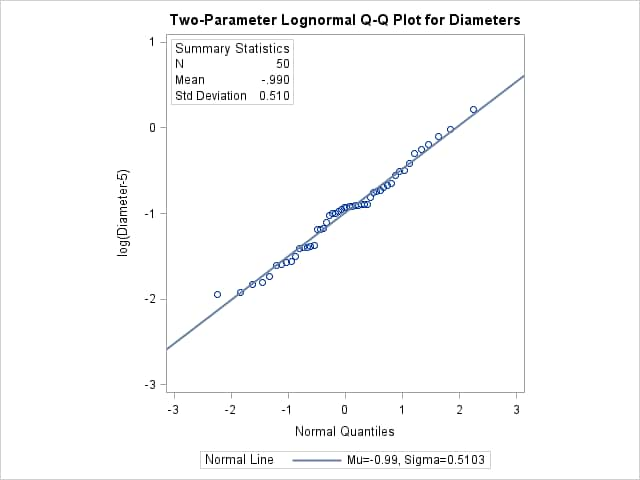# The UNIVARIATE Procedure

### Example 4.33 Estimating Parameters from Lognormal Quantile Plots

This example, which is a continuation of Example 4.31, demonstrates techniques for estimating the shape, location, and scale parameters, and the theoretical percentiles for a two-parameter lognormal distribution.

If the threshold parameter is known, you can construct a two-parameter lognormal Q-Q plot by subtracting the threshold from the data values and making a normal Q-Q plot of the log-transformed differences, as illustrated in the following statements:

```data ModifiedMeasures;
set Measures;
LogDiameter = log(Diameter-5);
label LogDiameter = 'log(Diameter-5)';
run;
```
```title 'Two-Parameter Lognormal Q-Q Plot for Diameters';
proc univariate data=ModifiedMeasures noprint;
qqplot LogDiameter / normal(mu=est sigma=est)
square
odstitle = title;
inset n mean (5.3) std (5.3) /
pos = nw header = 'Summary Statistics';
run;
```

Output 4.33.1: Two-Parameter Lognormal Q-Q Plot for DiametersBecause the point pattern in Output 4.33.1 is linear, you can estimate the lognormal parameters and as the normal plot estimates of and , which are –0.99 and 0.51. These values correspond to the previous estimates of –0.92 for and 0.5 for from Example 4.31. A sample program for this example, uniex18.sas, is available in the SAS Sample Library for Base SAS software.